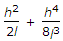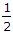# Civil Engineering - Surveying - Discussion

Discussion Forum : Surveying - Section 1 (Q.No. 2)
2.
If h is the difference in level between end points separated by l, then the slope correction is. The second term may be neglected if the value of h in a 20 m distance is less thanm
1 m
2 m
3 m
Explanation:
No answer description is available. Let's discuss.
Discussion:
67 comments Page 6 of 7.

Kawal jee v said:   7 years ago
@Vijay.

Rahul verman said:   8 years ago
How it will come 3.3?

Abhilash jana said:   8 years ago
When distances are measured along the slope, the equivalent horizontal distance may be determined by applying a slope correction.

The vertical slope angle of the length measured must be measured. (Refer to the figure on the other side).

Thus, For gentle slopes, m<20%.
Ch = h^2/2l.

For steep slopes, 20% <= m <= 30%.
Ch = h^2/2l+h^4/8l^3.

For very steep slopes, m>30%.
Ch = l(1-cos0).

For slopes greater than 5% the above formula must be adopted otherwise the second part of the formula can be neglected.

Since the first three options are less than or equal to 5% they can neglected as the 3m is greater than 5% the second part of formula must included. Hence the answer is (D) 3m.

Suparna said:   8 years ago
If h2 = 4O. Then h = 6.324.

How it will come 3.3? Please explain.

Rajesh said:   8 years ago
How it comes 3.3?

Rajesh said:   8 years ago
How it comes 3.3?

AKSHAY VASHISHT said:   8 years ago
Second term is neglected for slope less than 10%.

Slope for given problem for:

C) (2/20)*100 = 10%.
D) (3/20)*100 = 15%.

So for h = 2 m or less it is neglected.

And for h = 3 m it will be taken into account.

Raju said:   9 years ago
h square = 40.

Square root of 40 = 6.32 or 6.33.

How get it 3.3?

Siva said:   9 years ago
h2 = 40 root 40 = 6.32.

How can you get 3.3?